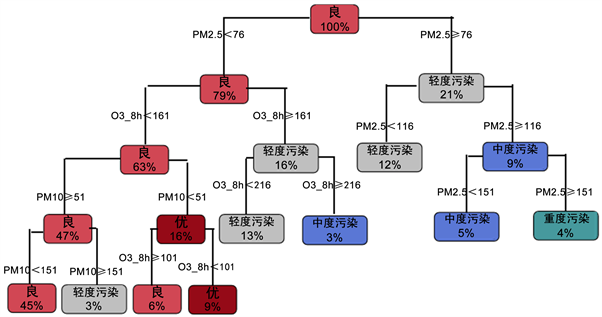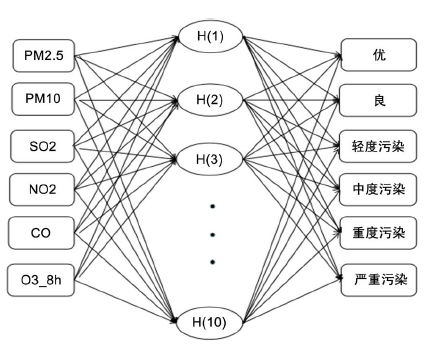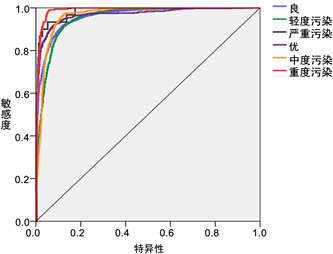﻿ 基于CART决策树和RBF神经网络的山东省空气污染状况预测评估

# 基于CART决策树和RBF神经网络的山东省空气污染状况预测评估 Prediction and Assessment of Air Pollution in Shandong Province Based on CART Decision Tree and Radial Basis Function Neural Network

Abstract: In order to better monitor air quality and make corresponding air protection measures, this paper uses CART tree to model the air quality level of Shandong Province in 2018, and the data from the first half of 2019 for classifying and predicting. Compared with RBF network, empirical analysis shows that the CART tree has a better fitting effect with higher model accuracy, and this model can also be applied to the forecasting and control of air pollution in Shandong Province.

1. 引言Table 1. AQI air quality classification

2. CART树原理

2.1. CART树

2.2. 分类树

2.2.1. Gini指数

1) 假设有K个类，样本点属于第K类的概率为 ${p}_{k}$，则概率分布的基尼指数定义为

$\text{Gini}\left(p\right)=\underset{k=1}{\overset{K}{\sum }}{p}_{k}\left(1-{p}_{k}\right)$ (1)

2) 对于二类分类问题，若样本点属于第1个类的概率为p，则概率分布的基尼指数为

$\text{Gini}\left(p\right)=p\left(1-p\right)$ (2)

3) 对于给定的样本集合D，其基尼指数为

$G\text{ini}\left(D\right)=1-\underset{k=1}{\overset{K}{\sum }}{\left(\frac{|{C}_{k}|}{|D|}\right)}^{2}$ (3)

${D}_{1}=\left\{\left(x,y\right)\in D|A\left(x\right)=a\right\},{D}_{2}=D-{D}_{1}$ (4)

$\text{Gini}\left(D,A\right)=\frac{|{D}_{1}|}{|D|}G\text{ini}\left({D}_{1}\right)+\frac{|{D}_{2}|}{|D|}\text{Gini}\left({D}_{2}\right)$ (5)

$\text{Gini}\left(D,A\right)$ 表示经 $A=a$ 分割后集合D的不确定性，基尼指数值越大，不确定性越大   。

2.2.2. CART树算法

1) 根据训练数据集D，从根结点开始，递归地对每个结点进行以下操作，构建二叉树：

2) 设结点的训练数据集为D，计算现有特征对该数据集的Gini系数。此时，对每一个特征A，对其可能取的每个值a，根据样本点对A = a的测试为“是”或“否”将D分割成 ${D}_{1}$${D}_{2}$ 两部分，计算A = a时的Gini系数。

3) 在所有可能的特征A以及它们所有可能的切分点a中，选择Gini系数最小的特征及其对应的切分点作为最优特征与最优切分点。依最优特征与最优切分点，从现结点生成两个子结点，将训练数据集依特征分配到两个子结点中去。

4) 对两个子结点递归地调用步骤l~2，直至满足停止条件。

5) 生成CART决策树。

2.3. 剪枝

1) 设 $k=0,T={T}_{0},\alpha =+\infty$

2) 自上而下地对各内部结点t计算 $C\left({T}_{t}\right),|{T}_{t}|$ 以及 $g\left(t\right)=\frac{C\left(T\right)-C\left({T}_{t}\right)}{|{T}_{t}|-1},\alpha =\mathrm{min}\left(\alpha ,g\left(t\right)\right)$ ；这里， ${T}_{t}$ 表示以t为根结点的子树， $C\left({T}_{t}\right)$ 是对训练数据的预测误差， $|{T}_{t}|$${T}_{t}$ 的叶节点个数；

3) 自上而下地访问内部结点t，如果有个 $g\left(t\right)=a$，进行剪枝，并对叶结点t以多数表决法决定其类，得到树T；

4) 设 $k=k+1,{\alpha }_{k}=\alpha ,{T}_{k}=T$

5) 如果T不是由根节点单独构成的树，则回到步骤(4)；

6) 采用交叉验证法在子树序列 ${T}_{0},{T}_{1},\cdots ,{T}_{n}$ 中选择最优子树 ${T}_{\alpha }$  -  。

3. 径向基神经网络

3.1. RBF神经网络

RBF神经网络的基本思想：用RBF作为隐单元的“基”构成隐藏层空间，隐藏层对输入矢量进行变换，将低维的模式输入数据变换到高维空间内，使得在低维空间内的线性不可分问题在高维空间内线性可分。就是用RBF的隐单元的“基”构成隐藏层空间，这样就可以将输入矢量直接(不通过权连接)映射到隐空间。当RBF的中心点确定以后，这种映射关系也就确定了。

3.2. RBF算法

1) 输入层X：由信号源节点构成，仅起到数据信息的传递作用，对输入信息不作任何变换。

2) 隐藏层H：节点数视需要而定. 隐含层神经元核函数(作用函数)是高斯函数，对输入信息进行空间映射的变换。

3) 输出层Y：对输入模式作出响应. 输出层神经元的作用函数为线性函数，对隐含层神经元输出的信息进行线性加权后输出，作为整个神经网络的输出结果。

${y}_{i}=\underset{i=1}{\overset{{n}_{c}}{\sum }}{w}_{i}g\left(‖x-{c}_{i}‖/{\sigma }_{i}\right)+b$ (6)

${R}_{i}\left(x\right)=\mathrm{exp}\left[-{‖x-{c}_{i}‖}^{2}/\left(2{\sigma }_{i}^{2}\right)\right]$ (7)

$Y=f\left(x\right)=WR={\sum }_{i=1}^{{n}_{c}}{w}_{i}{R}_{i}\left(x\right)$ (8)

4. 描述性统计Figure 1. Air quality category for each month

5. 预测模型

5.1. CART决策树

5.1.1. 模型建立Figure 2. CART-tree model

1) PM2.5、PM10和O3_8h是影响空气质量级别的主要因素。

2) 当 ${\text{PM}}_{2.5}\ge 151$ 时空气质量级别直接划分为重度污染；

3) ${\text{PM}}_{2.5}<151$$161\le {\text{O}}_{\text{3}}\text{_}8\text{h}<\text{215}\text{.5}$$116\le {\text{PM}}_{2.5}<156$ 时，空气质量级别划分为中度污染；

4) $76\le {\text{PM}}_{2.5}<116$${\text{PM}}_{2.5}<76$$161\le {\text{O}}_{\text{3}}\text{_}8\text{h}<\text{216}$${\text{PM}}_{2.5}<76$${\text{O}}_{\text{3}}\text{_}8\text{h}<\text{161}$$51\le {\text{PM}}_{10}<151$ 时，空气质量级别划分为轻度污染；

5) 当 ${\text{PM}}_{2.5}<76$${\text{O}}_{3}\text{_}8\text{h}<\text{161}$${\text{PM}}_{10}<151$${\text{PM}}_{2.5}<76$${\text{O}}_{3}\text{_}8\text{h}<\text{161}$${\text{O}}_{\text{3}}\text{_}8\text{h}\ge \text{101}$ 时，空气质量级别划分为良；

6) 当 ${\text{PM}}_{2.5}<76$${\text{O}}_{\text{3}}\text{_}8\text{h}<\text{101}$${\text{PM}}_{10}<51$ 时，空气质量级别划分为优。

5.1.2. 决策树的剪枝Table 2. CART - tree complexity parameter table

5.1.3. 决策树的模型预测

5.2. 径向基函数神经网络

5.2.1. 模型建立Figure 3. RBF neural network model

5.2.2. 模型评价Figure 4. ROC curveTable 4. Confusion matrix of training setTable 5. Confusion matrix of testing set

5.3. 模型优劣对比

CART树既可以做分类算法，也可以做回归。其优点为：1) 可以生成可以理解的规则。2) 计算量相对来说不是很大。3) 决策树可以清晰的显示哪些字段比较重要。缺点为：1) 当类别太多时，错误可能就会增加的比较快。2) 一般的算法分类的时候，只是根据一个字段来分类。

 Kampa, M. and Castanas, E. (2008) Human Health Effects of Air Pollution. Environmental Pollution, 151, 362-367.
https://doi.org/10.1016/j.envpol.2007.06.012

 Zhan, D.S., Kwan, M.-P., Zhang, W.Z., et al. (2018) The Driving Factors of Air Quality Index in China. Journal of Cleaner Production, 197, 1342-1351.
https://doi.org/10.1016/j.jclepro.2018.06.108

 张松林. CART分类与回归树方法介绍[J]. 火山地质与矿产, 1997(1): 67-75.

 Kim, B. and Kim, J. (2016) Stochastic Ordering of Gini Indexes for Multivariate Elliptical Risks. Insurance Mathematics and Economics, 68, 84-91.

 刘云翔, 吴浩. 基于改进CART决策树建立水华预警模型[J]. 中国农村水利水电, 2018(1): 26-28.

 蔡丽清. 基于CART算法的高校超市服务应用研究[J]. 电脑知识与技术, 2016, 12(13): 261-263.

 黄晓君. 基于变化检测CART决策树模式自动识别沙漠化信息[J]. 灾害学, 2017, 32(1): 36-42.

 孔颖. 基于CART算法的垃圾邮件过滤模型设计与实现[J]. 计算机应用, 2009, 29(2): 374-376.

 钱揖丽. 基于分类回归树CART的汉语韵律短语边界识别[J]. 计算机工程与应用, 2006, 44(6): 169-171.

 刘玉茹. CART分析及其在故障趋势预测中的应用[J]. 计算机应用, 2017(S2): 57-59.

 冯洁. CART算法在银行CRM中的应用研究[J]. 高效理科研究, 2011(26): 111-112.

 Shang, Z.G., Deng, T., He, J.Q. and Duan, X.H. (2019) A Novel Model for Hourly PM2.5 Concentration Prediction Based on CART and EELM. Sci-ence of the Total Environment, 651, 3043-3052.
https://doi.org/10.1016/j.scitotenv.2018.10.193

 Breiman, L., Friedman, J.H., Olshen, R.A. and Stone, C.J. (1984) Classification and Regression Trees, Wadsworth.

 Bai, Y., Li, Y., Wang, X.X., Xie, J.J., et al. (2016) Air Pollutants Concentrations Forecasting Using Back Propagation Neural Network Based on Wavelet Decomposition with Meteorological Conditions. Atmospheric Pollution Research, 7, 557-566.
https://doi.org/10.1016/j.apr.2016.01.004

 Zhu, S.L., Lian, X.Y., Liu, H.X., Hu, J.M., Wang, Y.Y. and Che, J.X. (2017) Daily Air Quality Index Forecasting with Hybrid Models: A Case in China. Environmental Pollution, 231, 1232-1244.
https://doi.org/10.1016/j.envpol.2017.08.069

 He, Q.F., Shahabi, H. and Shirzadi, A. (2019) Landslide Spatial Modelling Using Novel Bivariate Statistical Based Naïve Bayes, RBF Classifier, and RBF Network Machine Learning Algorithms. Science of the Total Environment, 663, 1-15.
https://doi.org/10.1016/j.scitotenv.2019.01.329

 Park, J. and Sandberg, I.W. (1993) Approximation and Radial-Basis-Function Networks. Neural Computation, 5, 305-316.
https://doi.org/10.1162/neco.1993.5.2.305

 Dong, J., Zhao, Y.X. and Liu, C. (2019) Orthogonal Least Squares Based Center Selection for Fault-Tolerant RBF Networks. Neurocomputing, 339, 217-231.
https://doi.org/10.1016/j.neucom.2019.02.039

Top